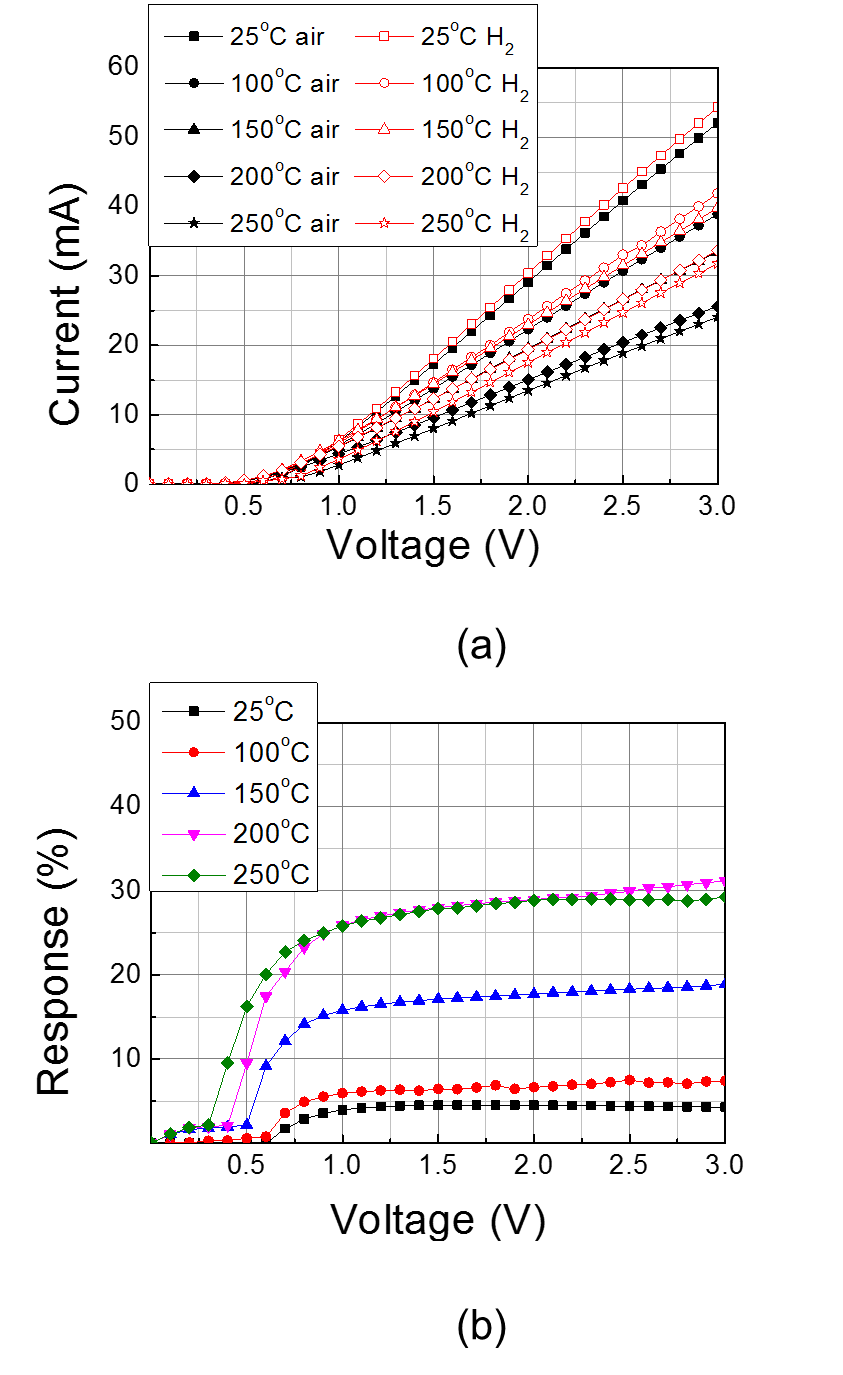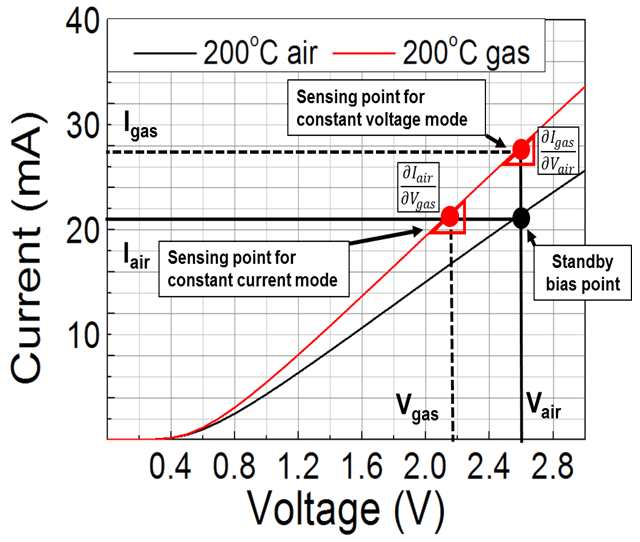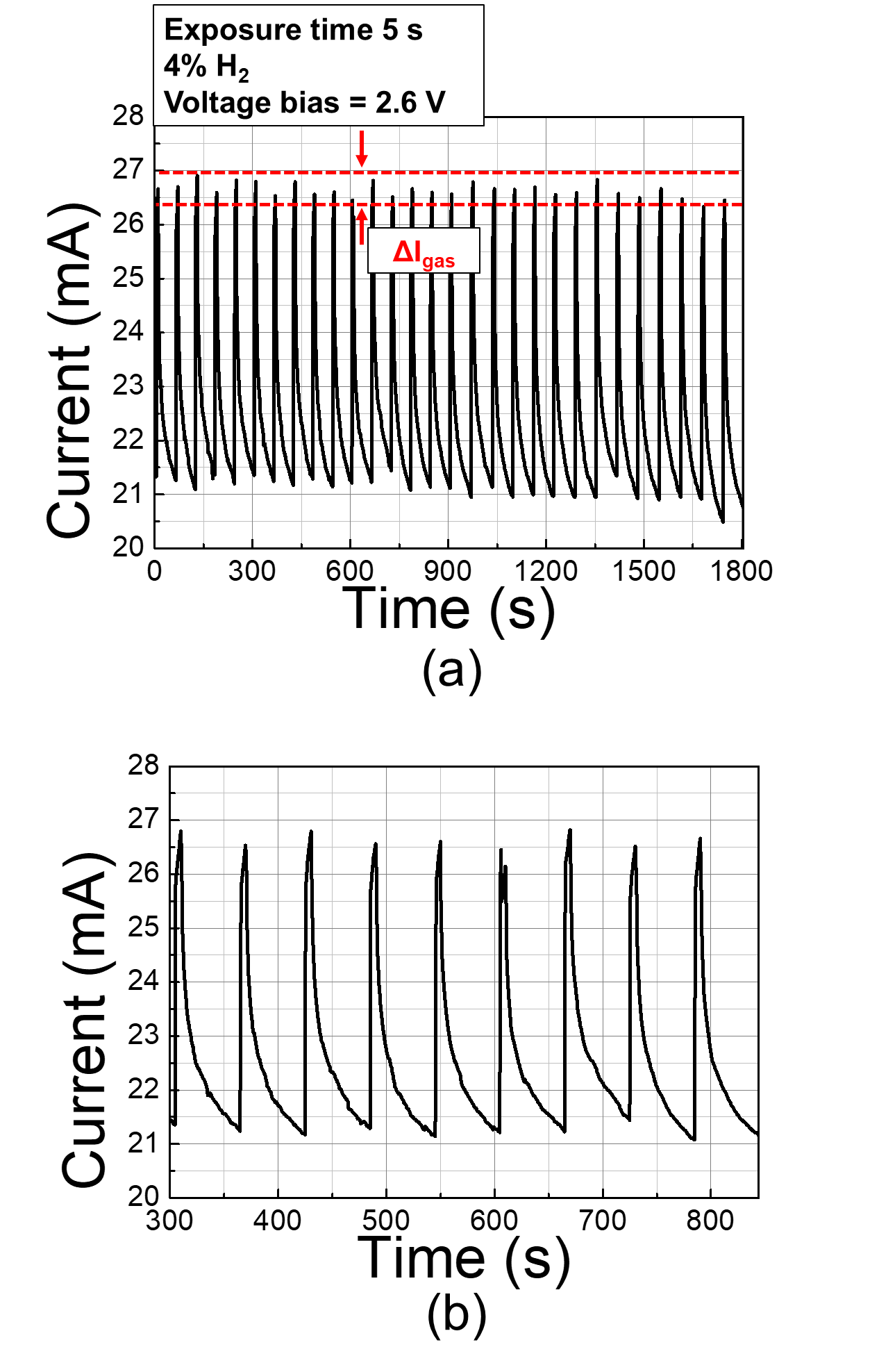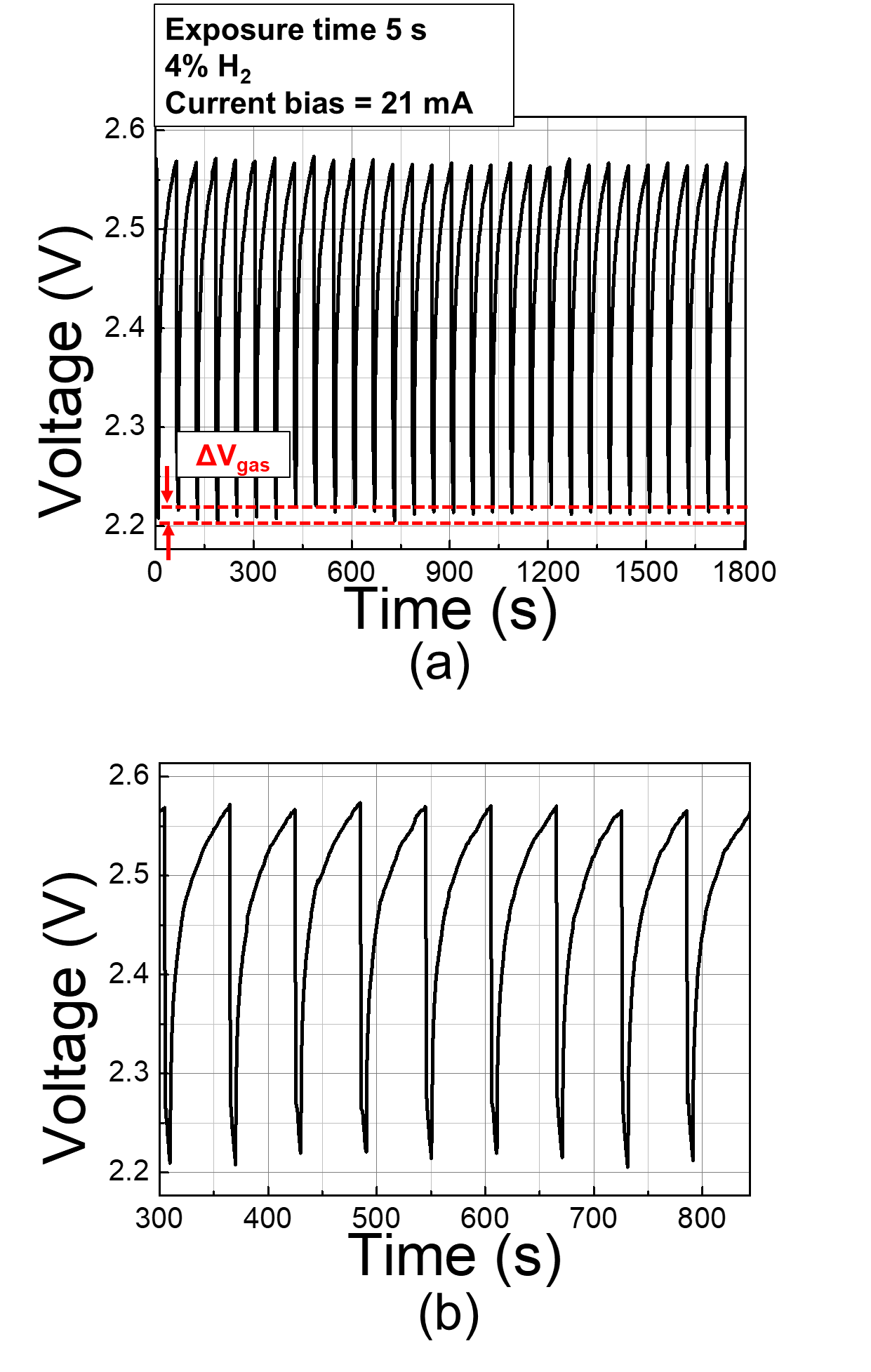Mobile QR CodeAlGaN/GaN heterojunction, Pd catalyst, Schottky-diode-type hydrogen sensor, stability, constant current bias mode

## I. INTRODUCTION

Schottky-diode-type hydrogen sensors were fabricated on an AlGaN/GaN-on-Si heterojunction platform using a Pd catalyst. Pd is considered a suitable catalyst for hydrogen detection owing to its high hydrogen adsorption and diffusion properties (9). The AlGaN/GaN heterojunction epitaxial structure comprises a 10-nm in-situ $\text{SiN}_\text{x}$ passivation layer, a 3.5 nm GaN cap layer, a 23 nm $Al_{0.24}Ga_{0.76}N$ barrier layer, and a 4.2 ${μ}$m GaN buffer layer grown on a (111)-oriented silicon substrate. The epitaxial wafer exhibited a two-dimensional electron gas (2DEG) concentration of 9 ${\times}$ 1012 cm$^{-2}$ and an electron mobility of 1,470 cm$^{2}$/Vs.

The fabrication process of the hydrogen sensor is as follows. After solvent cleaning, the cathode ohmic area was defined by photolithography, under which the in situ $\text{SiN}_\text{x}$, GaN cap, and AlGaN barrier layers were etched. The $\text{SiN}_\text{x}$ was etched using $CF_4$-based inductively coupled plasma-reactive ion etch (ICP-RIE), whereas the GaN and AlGaN layers were etched using $Cl_{2}/BCl_{3}$-based ICP-RIE. The AlGaN barrier layer was partially etched, leaving a 10 nm thick AlGaN layer. A Ti/Al/Ni/Au (= $200/1200/250/500$ Å) metal stack was then used for the ohmic contacts and was annealed at 830$^{\circ}$C for 30 s in an N2 ambient using a rapid thermal annealing process. The active area isolation was then performed by the same plasma etching recipe as that used for the ohmic contact process. The etch depth for isolation was ~350 nm. The measured contact resistance and sheet resistance were 0.81 ${\omega}$mm and 860 ${\omega}$/sq, respectively. The catalyst anode area of diameter 100 ${\mathrm{\mu}}$m was patterned and etched down to the middle of the AlGaN barrier layer using similar $CF_4$ and $Cl_{2}/BCl_{3}$-based etching recipes. The remaining AlGaN layer thickness was ~8 nm. The distance between the catalyst anode and the ohmic cathode was 5 ${μ}$m. A 30 nm thick Pd film was evaporated as the catalyst material. A Ti/Au (= 20/250 nm) metal stack was then used to deposit the pad electrodes, and a 100 nm thick $\text{SiN}_\text{x}$ film was deposited at 190$^{\circ}$C using a $SiH_{4}/N_{2}$ gas mixture and plasma-enhanced chemical vapor deposition. Finally, the catalyst window and electrode contact areas were etched with a $CF_4$-based plasma etching recipe. The microscopic top-view image and cross-sectional schematic of the fabricated AlGaN/GaN-on-Si hydrogen sensor and the transmission electron microscopy (TEM) cross-sectional image for the catalyst region are shown in Fig. 1(a)-(c), respectively.

## II. EXPERIMENTS AND DISCUSSION

### 1. Sensor Fabrication

Schottky-diode-type hydrogen sensors were fabricated on an AlGaN/GaN-on-Si heterojunction platform using a Pd catalyst. Pd is considered a suitable catalyst for hydrogen detection owing to its high hydrogen adsorption and diffusion properties (9). The AlGaN/GaN heterojunction epitaxial structure comprises a 10-nm in-situ $\text{SiN}_\text{x}$ passivation layer, a 3.5 nm GaN cap layer, a 23 nm $Al_{0.24}Ga_{0.76}N$ barrier layer, and a 4.2 ${μ}$m GaN buffer layer grown on a (111)-oriented silicon substrate. The epitaxial wafer exhibited a two-dimensional electron gas (2DEG) concentration of 9 ${\times}$ 1012 cm$^{-2}$ and an electron mobility of 1,470 cm$^{2}$/Vs.

The fabrication process of the hydrogen sensor is as follows. After solvent cleaning, the cathode ohmic area was defined by photolithography, under which the in situ $\text{SiN}_\text{x}$, GaN cap, and AlGaN barrier layers were etched. The $\text{SiN}_\text{x}$ was etched using $CF_4$-based inductively coupled plasma-reactive ion etch (ICP-RIE), whereas the GaN and AlGaN layers were etched using $Cl_{2}/BCl_{3}$-based ICP-RIE. The AlGaN barrier layer was partially etched, leaving a 10 nm thick AlGaN layer. A Ti/Al/Ni/Au (= $200/1200/250/500$ Å) metal stack was then used for the ohmic contacts and was annealed at 830$^{\circ}$C for 30 s in an N2 ambient using a rapid thermal annealing process. The active area isolation was then performed by the same plasma etching recipe as that used for the ohmic contact process. The etch depth for isolation was ~350 nm. The measured contact resistance and sheet resistance were 0.81 ${\omega}$mm and 860 ${\omega}$/sq, respectively. The catalyst anode area of diameter 100 ${\mathrm{\mu}}$m was patterned and etched down to the middle of the AlGaN barrier layer using similar $CF_4$ and $Cl_{2}/BCl_{3}$-based etching recipes. The remaining AlGaN layer thickness was ~8 nm. The distance between the catalyst anode and the ohmic cathode was 5 ${μ}$m. A 30 nm thick Pd film was evaporated as the catalyst material. A Ti/Au (= 20/250 nm) metal stack was then used to deposit the pad electrodes, and a 100 nm thick $\text{SiN}_\text{x}$ film was deposited at 190$^{\circ}$C using a $SiH_{4}/N_{2}$ gas mixture and plasma-enhanced chemical vapor deposition. Finally, the catalyst window and electrode contact areas were etched with a $CF_4$-based plasma etching recipe. The microscopic top-view image and cross-sectional schematic of the fabricated AlGaN/GaN-on-Si hydrogen sensor and the transmission electron microscopy (TEM) cross-sectional image for the catalyst region are shown in Fig. 1(a)-(c), respectively.

### 2. Sensor Characterization

Sensor characterization was conducted using a hydrogen gas concentration of 4% at various temperatures, i.e., 25$^{\circ}$C, 100$^{\circ}$C, 150$^{\circ}$C, 200$^{\circ}$C, and 250$^{\circ}$C. The current–voltage characteristics with and without hydrogen injection as a function of temperature are plotted in Fig. 2(a). The current levels were observed to increase with hydrogen injection regardless of the temperature, which was associated with the reduced surface potential caused by the Pd-H chemical reaction (10,11). The dipole effects of the hydrogen atoms lowered the surface potential of the AlGaN/GaN heterojunction, thereby enhancing the 2DEG concentration. This reaction is reversible and can be recovered to the initial state when hydrogen injection is terminated.

The hydrogen sensor response is defined by Eq. (1) (12).

##### (1)
$$\text {Response}=\frac{\left(I_{\text {gas}}-I_{\text {ar}}\right)}{I_{\text {air}}} \times 100$$

where Igas is the current measured with hydrogen injection and $I_{air}$ is the standby current without hydrogen injection. The calculated response characteristics as a function of temperature are shown in Fig. 2(b). The responses of the sensor were enhanced as the temperature increased up to 200oC, which was owing to the enhanced dissolution of the hydrogen atoms on the Pd surface (13). However, no further enhancement was observed at 250oC due to the saturated catalytic reaction. A maximum response of ~30% was achieved at 200$^{\circ}$C.

Fig. 2. (a) Current–voltage, (b) response characteristics of the fabricated Pd-functionalized AlGaN/GaN-on-Si diode-type hydrogen sensor with and without hydrogen injection as a function of temperature.Fig. 3. Constant voltage versus constant current modes. The standby bias point is at ($I_{air}$, $V_{air}$).### 3. Bias-dependent Stability Characteristics

To investigate the stability of the sensor signal, two different bias modes were tested, namely constant voltage and constant current bias modes. The sensor bias (i.e., standby condition) and sensing points are depicted in Fig. 3 whose current–voltage characteristics correspond to those at 200$^{\circ}$C in Fig. 2(a). When the sensor is operated in the constant voltage bias mode, the output current fluctuations as a function of the voltage bias fluctuation can be expressed by the derivative function $\left(\frac{\partial V_{g a s}}{\partial I_{a i r}}\right)$ at the sensing point ($I_{gas}, V_{air}$), which indicates the sensitivity with which the output current varies with the voltage bias fluctuation. When the fluctuation of the voltage supply is $\alpha V_{air}$, where is the fluctuation factor, the uncertainty of the output current can be defined as Eq. (2).

##### (2)
$$\frac{\Delta I_{g a s}}{\left|I_{g a s}-I_{a i r}\right|}=\alpha\left(\frac{\partial I_{g a s}}{\partial V_{a i r}}\right)\left(\frac{V_{a i r}}{\left|I_{g a s}-I_{a i r}\right|}\right)$$

Fig. 4. (a) Sensing repeatability characteristics of the fabricated Pd-functionalized recessed AlGaN/GaN-on-Si diode-type hydrogen sensor measured at 200$^{\circ}$C using a constant bias voltage of 2.6 V, (b) magnified time transient characteristics.Fig. 5. (a) Sensing repeatability characteristics of the fabricated Pd-functionalized recessed AlGaN/GaN-on-Si diode-type hydrogen sensor measured at 200$^{\circ}$C using a constant current bias of 21 mA, (b) magnified time transient characteristics.When the sensor is operated in the constant current bias mode, the output voltage fluctuations as a function of the current bias fluctuation can be expressed by the derivative function $\frac{\partial V_{gas}}{\partial I_{air}}$ at the sensing point ($V_{gas}, I_{air}$). When the fluctuation of the current supply is , the uncertainty of the output voltage can be defined as Eq. (3).

##### (3)
$$\frac{\Delta V_{g a s}}{\left|V_{g a s}-V_{a i r}\right|}=\alpha\left(\frac{\partial V_{g a s}}{\partial I_{a i r}}\right)\left(\frac{I_{a r r}}{\left|V_{g a s}-V_{a i r}\right|}\right)$$

The sensing repeatability tests were carried out at 200$^{\circ}$C with a hydrogen concentration of 4% using the two different bias operation modes, i.e., constant voltage and constant current operations. Hydrogen gas was first introduced for 5 s, then paused for 55 s, and the process was again repeated for 30 min. The standby bias point used for the repeatability tests was a bias voltage ($V_{air}$) of 2.6 V and bias current ($I_{air}$) of 21 mA.

Fig. 4(a) and (b) show the repeatability and magnified time transient characteristics measured using a constant voltage bias of 2.6 V. The derivative value at the sensing point for the constant voltage mode in Fig. 3 was obtained as $\frac{\partial V_{gas}}{\partial I_{air}}$ = 0.014~A/V, from which the uncertainty was calculated to be 6.26${\alpha}$.

Fig. 5(a) and (b) show the repeatability and time transient characteristics measured using a constant current bias of 21 mA. The derivative value at the sensing point for the constant current mode in Fig. 3 was obtained as $\frac{\partial V_{gas}}{\partial I_{air}}$ = 66.67~V/A, from which the uncertainty was calculated to be 3.92${\alpha}$.

Because the diode current has an exponential relationship with the voltage, the uncertainty in the constant voltage mode is higher than that in the constant current mode. Indeed, the constant current mode operation exhibited more stable output signal characteristics, as seen from Fig. 4 and 5. The fluctuation of the output signal in the constant voltage mode was $\frac{\Delta I_{g a s}}{\left|I_{g a s}-I_{a i r}\right|}$ = 0.05, whereas that in the constant current mode was $\frac{\Delta V_{g a s}}{\left|V_{g a s}-V_{a i r}\right|}$ = 0.016. Therefore, it is suggested that a constant current mode of operation can provide more accurate detection of hydrogen concentrations.

## IV. CONCLUSIONS

A Pd-functionalized hydrogen sensor was implemented using an AlGaN/GaN-on-Si heterojunction diode and investigated under different operation conditions, namely constant voltage and constant current bias modes. Since the diode current has an exponential relationship with the voltage, small fluctuations in the bias voltage tend to result in large variations in the output current. Therefore, it is suggested that a constant current bias mode of operation is more stable than the constant voltage mode.

### ACKNOWLEDGMENTS

This work was supported by Korea Electric Power Corporation (Grant: R18XA02) and Basic Science Research Programs (2015R1A6A1A03031833, 2016R1D1A1B03935445) through the National Research Foundation of Korea (NRF).

### REFERENCES

1
Debabrata Das, Sep 2009, Advances in biohydrogen production process: An approach towards commercialization, International Journal of Hydrogen Energy, Vol. 34, No. 17, pp. 7349-73572
Steinberg Meyer , Cheng Hsing C., Aug 1989, Modern and prospective technologies for hydrogen production from fossil fuels, International Journal of Hydrogen Energy, Vol. 14, No. 11, pp. 797-8203
Chung G., Cha H.-Y., Kim H., Jul 2018, Enhanced hydrogen sensitivity of AlGaN/GaN heterojunction gas sensors by GaN-cap layer, Electronics Letters, Vol. 54, No. 14, pp. 896-8974
Burk Jr. A.A., O’Loughlin M.J., Siergiej R.R., Agarwal A.K., Sriram S., Clarke R.C., MacMillan M.R., Balakrishna V., Brandt C.D., 1999, SiC and GaN wide bandgap semiconductor materials and devices, Solids-State Electronics, Vol. 43, No. 8, pp. 1459-14645
Chen Jr-Tai, Nilsson Daniel, Hsu Chih-Wei, Palisaitis Justinas, Forsberg Urban, Persson Per O.Å., Janzen and Erik, Jun 2015, Room-temperature mobility above 2200 cm2/V.s of two-dimensional electron gas in a sharp-interface AlGaN/GaN heterostructure, Applied Physics Letters, Vol. 106, No. 25, pp. 2516016
Choi June-Heang, Kim Hyungtak, Sung Hyuk-Kee, Cha Ho-Young, Dec 2019, Investigation of Stability and Power Consumption of an AlGaN/GaN Heterostructure Hydrogen Gas Sensor Using Different Bias Conditions, Sensors, Vol. 19, No. 24, pp. 55497
Choi J.-H., Jo M.-G., Han S.-W., Kim H., Kim S.-H., Jang S., Cha J.-S. Kim and H.-Y., Aug 2017, Hydrogen gas sensor of Pd-functionalised AlGaN/GaN heterostructure with high sensitivity and low-power consumption, Electronics Letters, Vol. 53, No. 17, pp. 1200-12028
Matsuo Kazushi, Negoro Noboru, Ktani Junji, Hashizume Tamotsu, Hasegawa Hideki, May 2005, Pt Schottky diode gas sensors formed on GaN and AlGaN/GaN heterostructure, Applied Surface Science, Vol. 244, No. 1-4, pp. 273-2769
Fisser Maximilian, Badcock Rodney A., Teal Paul D., Hunze Avid, Dec 2019, Optimizing the sensitivity of palladium based hydrogen sensors, Sensors and Actuatros B: Chemcial, Vol. 259, pp. 10-1910
Choi June-Heang, Vuong Tuan Anh, Kim Hyungtak, Cha Ho-Young, Jul 2020, High-Sensitivity Hydrogen Sensor Based on AlGaN/GaN Heterojunction Field-Effect transistor, Journal of Nanoscience and Nanotechnology, Vol. 20, No. 7, pp. 4404-440811
Safarik D.J., Schwarz R.B., Paglieri S.N., Quintana R.L., Tuggle D.G., Byler D.D., Sep 2009, Composition dependence of the elastic constants of β-phase and (α+β)-pahse PdHx, Ultrasonics, Vol. 50, No. 212
Kwang Hyeon Baik, Kim Jimin, Jang Soohwan, Jul 2017, Highly sensitive nonpolar a-plane GaN based hydrogen diode sensor with textured active area using photo-chemical etching, Sensors and Actuators B: Chemical, Vol. 238, pp. 462-46713
Khanuja M., Shrestha S., Mehta B. R., Kala S., Kruis E., Jul 2011, Magnitude and time response of electronic and topographical changes during hydrogen sensing in size selected palldium nanoparticles, Journal of Applied Physics, Vol. 110, No. 1, pp. 014318## Author

June-Heang Choi received B.S. degree in Materials Science & Engineering / Department of Chemical Engineering from Hongik University in Seoul, Korea, in 2015.

He received M.S. degree in Electronic and Electrical Engineering from Hongik University, Seoul, Korea, in 2018, respectively.

He is currently pursuing the Ph.D. degree at Hongik University.

His research interest is wide-bandgap semiconductor devices.

Hyungtak Kim received the B.S. degree in Electrical Engineering from Seoul National University, Seoul, Korea and the M.S./Ph.D. degree in Electrical and Computer Engineering from Cornell University, Ithaca, New York, U.S.A., in 1996 and 2003, respectively.

In 2007, he joined the school of electronic and electrical engineering at Hongik University, Seoul, Korea and is currently a professor.

His research interests include the reliability physics of semiconductor devices and those applications toward extreme environment electronics.

Prior to joining Hongik University, he spent 4 years developing CMOS devices and process integration for DRAM technology as a senior engineer in the semiconductor R&D center at Samsung Electronics, Co. Ltd.